SoftTree Technologies Technical Support Forums
 Register • Search • FAQ • Memberlist • Usergroups • Log in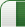FR: Custom Assistance for Spatial Functions in MySQL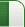Author Message
gemisigo

 Joined: 11 Mar 2010Posts: 1502Country: Hungary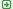FR: Custom Assistance for Spatial Functions in MySQL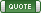I've tried to put together some info for Custom Assistance for Geometry and Spatial Functions in MySQL. It is certainly incomplete and might contain error here and there. Could you check them to see if they'd fit (and possibly fix them in case they don't)?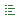Code:--Geometry Functions GeometryCollection(g1 geometry, g2 geometry, ...)GeometryCollection;Constructs a GeometryCollection. LineString(pt1 point, pt2 point, ...)LineString;Constructs a LineString value from a number of Point or WKB Point arguments. If the number of arguments is less than two, the return value is NULL. MultiLineString(ls1 linestring, ls2 linestring, ...)MultiLineString;Constructs a MultiLineString value using LineString or WKB LineString arguments. MultiPoint(pt1 point, pt2 point, ...)MultiPoint;Constructs a MultiPoint value using Point or WKB Point arguments. MultiPolygon(poly1, poly2, ...)MultiPolygon;Constructs a MultiPolygon value from a set of Polygon or WKB Polygon arguments. Point(x, y)Point;Constructs a Point using its coordinates. Polygon(ls1 linestring, ls2 linestring, ...)Polygon;Constructs a Polygon value from a number of LineString or WKB LineString arguments. If any argument does not represent a LinearRing (that is, not a closed and simple LineString geometry) - the return value is NULL. --Spatial Functions ST_GeomCollFromText(wkt varchar[, srid integer])GeometryCollection;Constructs a GeometryCollection value using its WKT representation and SRID. ST_GeometryCollectionFromText(wkt varchar[, srid integer])GeometryCollection;Constructs a GeometryCollection value using its WKT representation and SRID. ST_GeomCollFromTxt(wkt varchar[, srid integer])GeometryCollection;Constructs a GeometryCollection value using its WKT representation and SRID. ST_GeomFromText(wkt varchar[, srid integer])geometry;Constructs a geometry value of any type using its WKT representation and SRID ST_GeometryFromText(wkt varchar[, srid integer])geometry;Constructs a geometry value of any type using its WKT representation and SRID. ST_LineFromText(wkt varchar[, srid integer])LineString;Constructs a LineString value using its WKT representation and SRID. ST_LineStringFromText(wkt varchar[, srid integer])LineString;Constructs a LineString value using its WKT representation and SRID. ST_MLineFromText(wkt varchar[, srid integer])MultiLineString;Constructs a MultiLineString value using its WKT representation and SRID. ST_MultiLineStringFromText(wkt varchar[, srid integer])MultiLineString;Constructs a MultiLineString value using its WKT representation and SRID. ST_MPointFromText(wkt varchar[, srid integer])MultiPoint;Constructs a MultiPoint value using its WKT representation and SRID. ST_MultiPointFromText(wkt varchar[, srid integer])MultiPoint;Constructs a MultiPoint value using its WKT representation and SRID. ST_MPolyFromText(wkt varchar[, srid integer])MultiPolygon;Constructs a MultiPolygon value using its WKT representation and SRID. ST_MultiPolygonFromText(wkt varchar[, srid integer])MultiPolygon;Constructs a MultiPolygon value using its WKT representation and SRID. ST_PointFromText(wkt varchar[, srid integer])Point;Constructs a Point value using its WKT representation and SRID. ST_PolyFromText(wkt varchar[, srid integer])Polygon;Constructs a Polygon value using its WKT representation and SRID. ST_PolygonFromText(wkt varchar[, srid integer])Polygon;Constructs a Polygon value using its WKT representation and SRID. ST_X(point)double;Returns the X-coordinate value for the Point object p as a double-precision number. ST_Y(point)double;Returns the Y-coordinate value for the Point object p as a double-precision number. --Geometry Format Conversion Functions ST_AsBinary(g geometry)binary;Converts a value in internal geometry format to its WKB representation and returns the binary result. ST_AsText(g geometry)string;Converts a value in internal geometry format to its WKT representation and returns the string result. ST_AsWKB(g geometry)binary;Converts a value in internal geometry format to its WKB representation and returns the binary result. ST_AsWKT(g geometry)string;Converts a value in internal geometry format to its WKT representation and returns the string result. -- Spatial Operator Functions ST_Buffer(g, d[, strategy1[, strategy2[, strategy3]]])geometry;Returns a geometry that represents all points whose distance from the geometry value g is less than or equal to a distance of d, or NULL if any argument is NULL. The SRID of the geometry argument must be 0 because ST_Buffer() supports only the Cartesian coordinate system. If any geometry argument is not a syntactically well-formed geometry, an ER_GIS_INVALID_DATA error occurs. ST_Buffer_Strategy(strategy[, points_per_circle])strategy_byte_string;This function returns a strategy byte string for use with ST_Buffer() to influence buffer computation. The result is NULL if any argument is NULL. If any argument is invalid, an ER_WRONG_ARGUMENTS error occurs. ST_ConvexHull(g geometry)geometry;Returns a geometry that represents the convex hull of the geometry value g. ST_Difference(g1 geometry, g2 geometry)geometry;Returns a geometry that represents the point set difference of the geometry values g1 and g2. ST_Intersection(g1 geometry, g2 geometry)geometry;Returns a geometry that represents the point set intersection of the geometry values g1 and g2. ST_SymDifference(g1 geometry, g2 geometry)geometry;Returns a geometry that represents the point set symmetric difference of the geometry values g1 and g2, which is defined as: ST_Union(g1 geometry, g2 geometry)geometry;Returns a geometry that represents the point set union of the geometry values g1 and g2. --Spatial GeoJSON Functions ST_AsGeoJSON(g geometry [, max_dec_digits integer [, options integer]])GeoJSON:Generates a GeoJSON object from the geometry g. The object string has the connection character set and collation. ST_GeomFromGeoJSON(str [, options integer [, srid integer]])geometry;Parses a string str representing a GeoJSON object and returns a geometry. --Spatial Convenience Functions ST_Distance_Sphere(g1 geometry, g2 geometry [, radius])spherical_distance;Returns the mimimum spherical distance between two points and/or multipoints on a sphere, in meters, or NULL if any geometry argument is NULL or empty. ST_MakeEnvelope(pt1 point, pt2 point)rectangle;Returns the rectangle that forms the envelope around two points, as a Point, LineString, or Polygon. If any argument is NULL, the return value is NULL. ST_Simplify(g geometry, max_distance double)geometry;Simplifies a geometry using the Douglas-Peucker algorithm and returns a simplified value of the same type. If any argument is NULL, the return value is NULL. ST_Validate(g geometry)integer;Validates a geometry according to the OGC specification. ST_Validate() returns the geometry if it is a valid geometry byte string and is geometrically valid, NULL if the argument is not a valid geometry byte string or is not geometrically valid or is NULL. --Spatial Relation Functions That Use Object Shapes ST_Contains(g1 geometry, g2 geometry)integer;Returns 1 or 0 to indicate whether g1 completely contains g2. This tests the opposite relationship as ST_Within(). ST_Crosses(g1 geometry, g2 geometry)integer;The term spatially crosses denotes a spatial relation between two given geometries that has the following properties: ST_Disjoint(g1 geometry, g2 geometry)integer;Returns 1 or 0 to indicate whether g1 is spatially disjoint from (does not intersect) g2. ST_Distance(g1 geometry, g2 geometry)integer;Returns the distance between g1 and g2. If either argument is NULL or an empty geometry, the return value is NULL. ST_Equals(g1 geometry, g2 geometry)integer;Returns 1 or 0 to indicate whether g1 is spatially equal to g2. ST_Intersects(g1 geometry, g2 geometry)integer;Returns 1 or 0 to indicate whether g1 spatially intersects g2. ST_Overlaps(g1 geometry, g2 geometry)integer;Two geometries spatially overlap if they intersect and their intersection results in a geometry of the same dimension but not equal to either of the given geometries. ST_Touches(g1 geometry, g2 geometry)integer;Two geometries spatially touch if their interiors do not intersect, but the boundary of one of the geometries intersects either the boundary or the interior of the other. ST_Within(g1 geometry, g2 geometry)integer;Returns 1 or 0 to indicate whether g1 is spatially within g2. This tests the opposite relationship as ST_Contains(). --LineString and MultiLineString Property Functions ST_EndPoint(ls)Point;Returns the Point that is the endpoint of the LineString value ls. ST_IsClosed(ls)integer;For a LineString value ls, ST_IsClosed() returns 1 if ls is closed (that is, its ST_StartPoint() and ST_EndPoint() values are the same). For a MultiLineString value ls, ST_IsClosed() returns 1 if ls is closed (that is, the ST_StartPoint() and ST_EndPoint() values are the same for each LineString in ls). ST_Length(ls)double;Returns a double-precision number indicating the length of the LineString or MultiLineString value ls in its associated spatial reference. The length of a MultiLineString value is equal to the sum of the lengths of its elements. ST_NumPoints(ls)integer;Returns the number of Point objects in the LineString value ls. ST_StartPoint(ls)Point;Returns the Point that is the start point of the LineString value ls.

Sat Jul 15, 2017 5:45 am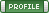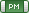SysOp

 Joined: 26 Nov 2006Posts: 6786Thank you for your contribution. Much appreciated. I will submit it for review and addition to the factory default settings.
Sat Jul 15, 2017 7:44 amgemisigo

 Joined: 11 Mar 2010Posts: 1502Country: HungaryYou're welcome. There'll be some details that will have to be taken care of eg. which versions it applies to. I guess it's 5.7.x for spatial function, where some variants without the ST_ prefix got deprecated and I've got absolutely no idea when the json functions came into picture. So there's still a lot to chew on this to make it work, but it might be a good start.
Sat Jul 15, 2017 7:55 amDisplay posts from previous: All Posts1 Day7 Days2 Weeks1 Month3 Months6 Months1 Year Oldest FirstNewest First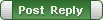All times are GMT - 4 Hours Page 1 of 1

 Jump to: Select a forum ----------------SQL AssistantDB Audit, DB Mail, DB Tools24x7 Scheduler, Event Server, Automation SuiteTips & Snippets Repository
You cannot post new topics in this forum
You cannot reply to topics in this forum
You cannot edit your posts in this forum
You cannot delete your posts in this forum
You cannot vote in polls in this forum